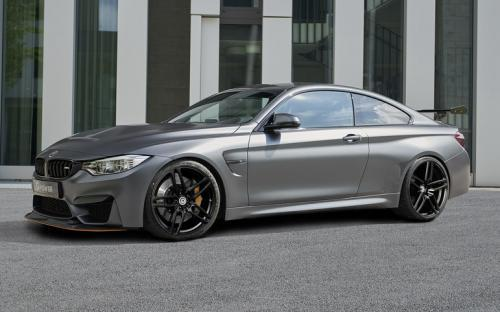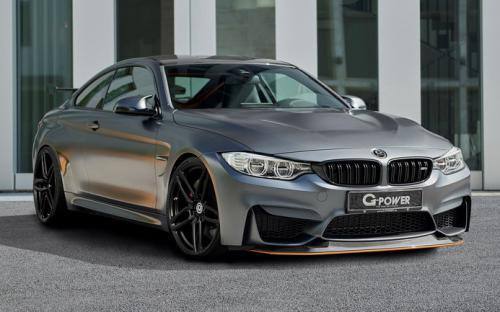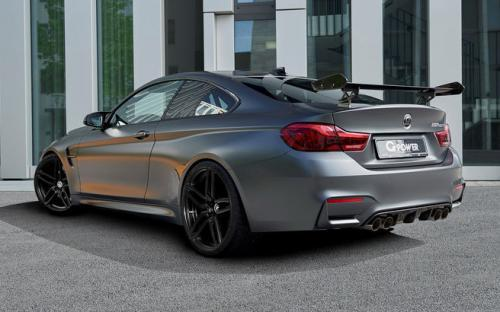### 宝马M 宝马M40 种颜色可选2016款最低售价：111.38 万元起

4689(mm)1870(mm)1390(mm)##### 配置亮点：
• 胎压监测装置

• ISOFIX儿童座椅接口

• 车身稳定控制(ESC/ESP/DSC等)

• 电动天窗

• 定速巡航

• 后倒车雷达

• 真皮座椅

• GPS导航系统

• 氙气大灯

• 后视镜加热

• 提交
2016款 M4双门轿跑车 竞速限量版 (156张)
• 2016款 M4双门轿跑车 竞速限量版 (156张)
• 2016款 M4 GTS (269张)
• 宝马M 宝马M4 绕车实拍• 宝马M 宝马M4 在售车型

排量 车型 厂商指导价 本地最低报价 购车工具
3.0L
M4双门轿跑车 7挡双离合
102.70万
102.70万

M4敞篷轿跑车 7挡双离合
113.00万
113.00万

### 宝马M 宝马M4 动力加速

宝马M4 0-100公里加速时间分布在 3.8-4.4秒 属于 超跑级

动力级别 加速时间 车型
高性能级(3款)
4.1s
M4双门轿跑车
4.4s
M4敞篷轿跑车
4.3s
M4敞篷轿跑车 竞速限量版M4双门轿跑车 MT
超跑级(2款)
4.0s
M4双门轿跑车 竞速限量版
3.8s
M4 GTS

宝马M 宝马M4 视频

宝马M 宝马M4 新闻资讯

# 宝马M3/M4竞速限量版上市 111.08万起

上市新车 超过8742次关注

据悉宝马官方宣布，M3/M4系列两款竞速限量版车型正式上市，其中M3竞速限量版售价111.08万元，M4竞速限量版售111.38-120.68万元；本次上市的三款车型分别限量推出50...

# 庆成立25周年 宝马M4纪念版官图发布

国产新车 超过9964次关注

为了纪念宝马个性化定制部门（Individualcustomizationdivision）成立25周年，宝马正式推出了由该部门打造的一款M4特别版车型。

# 换装增压套件 550马力版改装宝马M4

改装 超过3872次关注

作为欧洲大陆最大的改装展会，德国埃森改装展上自然不会少本土厂家的改装案例。作为德国知名的改装厂商Manhart在展会上带来一款基于宝马M4改装而来的展车。

# Hamann操刀改装517马力宝马M4敞篷版

新闻 超过3233次关注

豪车改装专家Hamann公司近日推出了一辆宝马M4敞篷版改装案例，为这辆车改装了一套包围套件和轮圈，同时动力也有所提升。

# 宝马M4限量版上市 限10台/售97.6万

进口新车 超过5801次关注

10月17日，宝马宣布在中国市场推出M4马年限量版，其特点在于配备了6速手动变速箱。据悉，该车将限量销售10台，售价为97.6万元。

# 抢先拍宝马M4双门概念车 国内首次亮相

汽车导购 超过8731次关注

从去年12月12日官图发布，到今年1月北美车展上的实车亮相，再到如今M4概念车在国内亮相，宝马M4这款备受瞩目的新车正在加快着其进入中国市场的步伐。今天给大家拍摄...

# 宝马新M3/M4接受预定 预售100万/105万

新闻 超过0次关注

日前，宝马官方宣布，宝马全新M3、M4车型已在国内接受预定，预售价依次为100万元、105万元(按照一般定价规律，不排除未来最终车型的指导价格比目前的预售价更低的可...

新闻 超过6299次关注

# 雨天赛道试驾宝马全新M3/M4 瑕不掩瑜

试驾评测 超过8390次关注

9月12日，也就是全新M3、M4上市的前一天，我终于有幸坐进了这两款车的驾驶舱，在上海F1赛车场感受这台3.0T双涡轮增压发动机的澎湃动力。不过从之前各家海外媒体的试...

# 宝马M3/M4于今日上市 预售100-105万

进口新车 超过5637次关注

宝马全新M3、M4以及M4敞篷版即将于8月21日上市销售（预计今日20:00左右公布价格），它们最大的变化是不再使用自然吸气发动机，而是全面更换3.0T涡轮增压发动机。根...

猜你喜欢

﻿
• 快速找车
• 选择品牌
• 选择品牌
• A  奥迪
• A  阿斯顿·马丁
• A  阿尔法·罗密欧
• B  宝沃
• B  布加迪
• B  巴博斯
• B  保时捷
• B  宾利
• B  奔驰
• B  宝马
• B  本田
• B  别克
• B  标致
• B  比亚迪
• B  宝骏
• B  北汽制造
• B  北汽新能源
• B  北汽幻速
• B  北汽威旺
• B  北京汽车
• B  奔腾
• B  北汽绅宝
• C  长安
• C  长安商用
• C  长城
• C  昌河
• D  大众
• D  道奇
• D  DS
• D  东南
• D  东风风神
• D  东风风行
• D  东风小康
• D  东风风度
• D  东风
• F  福特
• F  丰田
• F  菲亚特
• F  法拉利
• F  福田
• F  福迪
• F  福汽启腾
• G  观致
• G  广汽传祺
• G  广汽吉奥
• G  GMC
• H  红旗
• H  汉腾汽车
• H  哈弗
• H  哈飞
• H  海格
• H  海马
• H  华颂
• H  黄海
• H  华泰
• H  恒天
• J  吉利汽车
• J  捷豹
• J  Jeep
• J  江淮
• J  江铃
• J  金杯
• J  九龙
• J  金旅
• K  凯翼
• K  凯迪拉克
• K  克莱斯勒
• K  科尼塞克
• K  卡威
• K  开瑞
• L  路虎
• L  林肯
• L  劳斯莱斯
• L  兰博基尼
• L  雷克萨斯
• L  铃木
• L  雷诺
• L  理念
• L  力帆
• L  莲花汽车
• L  猎豹
• L  路特斯
• L  陆风
• M  马自达
• M  MG
• M  MINI
• M  玛莎拉蒂
• M  摩根
• M  迈凯轮
• N  纳智捷
• O  欧宝
• O  讴歌
• O  欧朗
• Q  奇瑞
• Q  起亚
• Q  启辰
• R  日产
• R  荣威
• R  瑞麒
• S  三菱
• S  斯威汽车
• S  萨博
• S  smart
• S  斯柯达
• S  斯巴鲁
• S  思铭
• S  双龙
• S  上汽大通
• S  双环
• T  特斯拉
• T  腾势
• W  沃尔沃
• W  五菱汽车
• W  五十铃
• W  威兹曼
• W  威麟
• X  现代
• X  雪佛兰
• X  雪铁龙
• X  西雅特
• Y  一汽
• Y  英菲尼迪
• Y  英致
• Y  依维柯
• Y  野马汽车
• Y  永源
• Z  众泰
• Z  中华
• Z  中兴
• Z  知豆
• 选择车系
• 选择车系
• 车型对比
• 选择品牌
• 选择品牌
• A  奥迪
• A  阿斯顿·马丁
• A  阿尔法·罗密欧
• B  宝沃
• B  布加迪
• B  巴博斯
• B  保时捷
• B  宾利
• B  奔驰
• B  宝马
• B  本田
• B  别克
• B  标致
• B  比亚迪
• B  宝骏
• B  北汽制造
• B  北汽新能源
• B  北汽幻速
• B  北汽威旺
• B  北京汽车
• B  奔腾
• B  北汽绅宝
• C  长安
• C  长安商用
• C  长城
• C  昌河
• D  大众
• D  道奇
• D  DS
• D  东南
• D  东风风神
• D  东风风行
• D  东风小康
• D  东风风度
• D  东风
• F  福特
• F  丰田
• F  菲亚特
• F  法拉利
• F  福田
• F  福迪
• F  福汽启腾
• G  观致
• G  广汽传祺
• G  广汽吉奥
• G  GMC
• H  红旗
• H  汉腾汽车
• H  哈弗
• H  哈飞
• H  海格
• H  海马
• H  华颂
• H  黄海
• H  华泰
• H  恒天
• J  吉利汽车
• J  捷豹
• J  Jeep
• J  江淮
• J  江铃
• J  金杯
• J  九龙
• J  金旅
• K  凯翼
• K  凯迪拉克
• K  克莱斯勒
• K  科尼塞克
• K  卡威
• K  开瑞
• L  路虎
• L  林肯
• L  劳斯莱斯
• L  兰博基尼
• L  雷克萨斯
• L  铃木
• L  雷诺
• L  理念
• L  力帆
• L  莲花汽车
• L  猎豹
• L  路特斯
• L  陆风
• M  马自达
• M  MG
• M  MINI
• M  玛莎拉蒂
• M  摩根
• M  迈凯轮
• N  纳智捷
• O  欧宝
• O  讴歌
• O  欧朗
• Q  奇瑞
• Q  起亚
• Q  启辰
• R  日产
• R  荣威
• R  瑞麒
• S  三菱
• S  斯威汽车
• S  萨博
• S  smart
• S  斯柯达
• S  斯巴鲁
• S  思铭
• S  双龙
• S  上汽大通
• S  双环
• T  特斯拉
• T  腾势
• W  沃尔沃
• W  五菱汽车
• W  五十铃
• W  威兹曼
• W  威麟
• X  现代
• X  雪佛兰
• X  雪铁龙
• X  西雅特
• Y  一汽
• Y  英菲尼迪
• Y  英致
• Y  依维柯
• Y  野马汽车
• Y  永源
• Z  众泰
• Z  中华
• Z  中兴
• Z  知豆
• 选择车系
• 选择车系
• 选择车型
• 选择车型
• 意见反馈